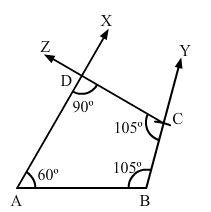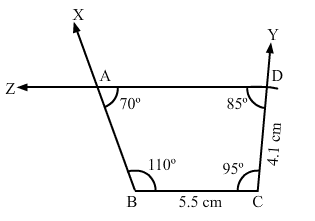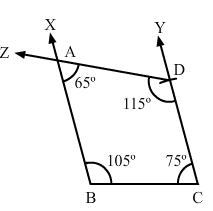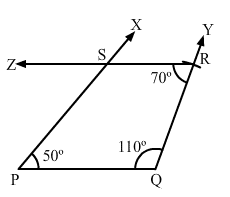×#### Thank you for registering.

One of our academic counsellors will contact you within 1 working day.

Click to Chat

1800-1023-196

+91-120-4616500

CART 0

• 0

MY CART (5)

Use Coupon: CART20 and get 20% off on all online Study Material

ITEM
DETAILS
MRP
DISCOUNT
FINAL PRICE
Total Price: Rs.

There are no items in this cart.
Continue Shopping• Complete JEE Main/Advanced Course and Test Series
• OFFERED PRICE: Rs. 15,900
• View Details

Practical Geometry Exercise 18.5

Question: 1

Construct a quadrilateral ABCD given that AB = 4 cm, BC = 3 cm, ∠A = 75°, ∠B = 80° and ∠C = 120°.

Solution:Steps of construction:

Step I: Draw AB = 4 cm.

Step II: Construct ∠XAB = 75° at A and ∠ABY = 80° at B.

Step III: With B as the center and radius 3 cm, cut off BC = 3 cm.

Step IV: At C, draw ∠BCZ = 120° such that it meets AX at D.

Question: 2

Construct a quadrilateral ABCD, where AB = 5.5 cm, BC = 3.7 cm, ∠A = 60°, ∠B=105° and ∠D = 90°.

Solution:We know that the sum of all the angles in a quadrilateral is 360.

i.e. ∠A + ∠B + ∠C + ∠D = 360°

∠C = 105°

Steps of construction:

Step I: Draw AB = 5.5 cm.

Step II: Construct ∠XAB=60° at A and ∠ABY=105°.

Step III: With B as the center and radius 3.7 cm, cut off BC = 3.7 cm

Step IV: At C, draw ∠BCZ = 105° such that it meets AX at D.

Question: 3

Construct a quadrilateral PQRS, where PQ = 3.5 cm, QR = 6.5 cm, ∠P = ∠R = 105° and ∠S = 75°.

Solution:We know that the sum of all the angles in a quadrilateral is 360.

i.e., ∠P + ∠Q + ∠R + ∠S = 360°

∠Q = 75°

Steps of construction:

Step I: Draw PQ = 3.5 cm.

Step II: Construct ∠XPQ = 75° at P and ∠PQY = 75° at Q.

Step III: With Q as the center and radius 6.5 cm, cut off QR = 6.5

Step IV: At R, draw ∠QRZ = 105° such that it meets PX at S.

Question: 4

Construct a quadrilateral ABCD when BC = 5.5 cm, CD = 4.1 cm, ∠A = 70°, ∠B = 110° and ∠D = 85°.

Solution:We know that the sum of all the angles in a quadrilateral is 360. i.e.

i.e. ∠A + ∠B + ∠C + ∠D = 360°

∠C = 95°

Steps of construction:

Step I: Draw BC = 5.5 cm.

Step II: Construct ∠XBC=110° at A and ∠BCY= 95°.

Step III: With C as the center and radius 4.1 cm, cut off CD = 4.1 cm.

Step IV: At D, draw ∠CDZ = 85° such that it meets BY at A.

Question: 5

Construct a quadrilateral ABCD, where ∠A = 65°, ∠B = 105°, ∠C = 75°, BC = 5.7 cm and CD = 6.8 cm.

Solution:We know that the sum of all the angles in a quadrilateral is 360. i.e

i.e. ∠A + ∠B + ∠C + ∠D = 360°

∠D = 115°

Steps of Construction:

Step I: Draw BC = 5.7 cm.

Step II: Construct ∠XBC = 105° at B and ∠BCY = 105° at C.

Step III: With C as the center and radius 6.8 cm, cut off CD = 6.8 cm.

Step IV: At D, draw ∠CDZ = 115° such that it meets BY at A.

Question: 6

Construct a quadrilateral PQRS, in which PQ = 4 cm, QR = 5 cm, ∠P = 50°, ∠Q = 110° and ∠R = 70°.

Solution:Steps of construction:

Step I: Draw PQ = 4 cm.

Step II: Construct ∠XPQ = 50° at P and ∠PQY = 110° at Q.

Step III: With Q as the center and radius 5 cm, cut off QR = 5 cm.

Step IV: At R, draw ∠QRZ = 70° such that it meets PX at S.### Course Features

• 728 Video Lectures
• Revision Notes
• Previous Year Papers
• Mind Map
• Study Planner
• NCERT Solutions
• Discussion Forum
• Test paper with Video Solution